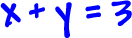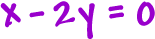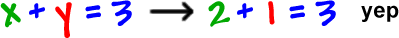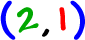Systems, 2 x 2 in this case, are when you have 2 equations and 2 unknowns (letters).

Here's an example:The goal is to find an x guy and a y guy that work in both equations.

In this chapter, I'll show you three different ways to solve these.

The first method is graphing.  This is a cool method to start with since it lets you see what's going on.

We've got two equations -- and they are equations of lines.  Let's graph them both on the same axes and see what we get.Hey, the two lines intersect at the point ( 2 , 1 ) . This means that ( 2 , 1 ) is a point in BOTH lines... So, x = 2 and y = 1 will work in the equations!So, the answer is.

Checking your answers, like we did at the end there, is a really good idea... and something you should always do on a test!  We're going to do it on every single problem we do!